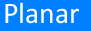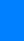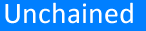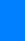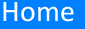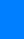• Renato Calleja, IIMAS, UNAM (Mexico)
• Eusebius Doedel, Department of Computer Science, Concordia University (Canada)
• Carlos García-Azpeitia, Facultad de Ciencias, UNAM (Mexico)

# Introduction

We continue numerically global Lyapunov families appearing from polygonal relative equilibria of the n-body problem in a rotating frame of reference. When the frequencies of the Lyapunov families and the rotating frame maintain a rational relation then the  Lyapunov orbits are periodic solutions in the inertial frame. We prove that a dense set of Lyapunov orbits with frequencies satisfying a Diophantine equation are choreographies. We present and discuss a sample of choreographies computed numerically along the Lyapunov families for n = 4,...,9.

Below is an example of a spatial choreography with 5 bodies.

 Note: if your browser is unable to see the animation above, please enable WebGL. Instructions HERE
The study of $n$ equal masses following the same path (choreographies) has gained a lot of attention in recent years. In , C. Moore (1993) found numerically a choreography where three bodies follow one another around a figure eight by minimizing the action among symmetric paths. Independently in , Chenciner and Montgomery (2000) gave a rigorous mathematical proof of the existence of this orbit by, minimizing the action over paths that connect a colinear and an isosceles configuration.

The results in  mark the beginning of the development of variational methods, where the existence of choreographies can be associated to the problem of finding critical point of the classical action of Newton equations of motion. The main obstacle encountered in the application of the principle of least action are the existence of paths with collisions, and the lack of compactness of the action. In , Terracini and Ferrero (2004) applied the principle of least action systematically over symmetric paths to avoid collisions, using ideas introduced by Marchal . For the discussion of these and other variational approaches see [3, 2, 11, 12, 28] and references therein. When the frequency varies along the vertical Lyapunov families then an infinite number of choreographies exists; a fact established in  for orbits close to the
polygon equilibrium, with $n \leq 6$.

Below is an example of a spatial choreography with 9 bodies.

 Note: if your browser is unable to see the animation above, please enable WebGL. Instructions HERE

Another procedure to obtain choreographies is by using continuation methods. Chenciner and Féjoz (2009) in  pointed out that choreographies appear in dense sets along the vertical Lyapunov families arising from $n$ bodies rotating in a polygon, see also [5, 15, 21]. The local existence of the vertical Lyapunov families is proven in  using Weinsten-Moser theory. While similar computations can be carried out for other values of $n$, a general analytical proof that is valid for all n remains an open problem.

Let $(u_{j},z_{j})\in\mathbb{C}\times\mathbb{R}$ be the positions of $n$ bodies of unitary masses in a rotating frame. The frequency of the rotation frame can be chosen such that the unitary polygon \begin{equation} u_{j}=e^{ij\zeta},\qquad z_{j}=0\text{,}\label{RE}  \end{equation} is equilibrium of the Newton's equations. The Lyapunov families emanate with frequencies equal to the natural modes of oscillation of the polygonal equilibrium. García-Azpeitia and J. Ize (2013) in  prove the global existence of the bifurcations of planar and vertical Lyapunov families using the equivariant degree theory in :

Theorem on planar Lyapunov families, : For $n\geq7$, for each integer $k$ such that $2\leq k\leq n-2,$ the polygonal relative equilibrium has one global bifurcation of planar periodic solutions with symmetries \begin{equation} u_{j}(t)=e^{ij\zeta}u_{n}(t+jk\zeta),\qquad u_{n}(t)=\bar{u}_{n} (-t)\text{.}\tag{PS}\label{PS} \end{equation}

Theorem on spatial Lyapunov families, : For $n\geq3$, for each $k$ such that $1\leq k\leq n/2,$ the polygonal relative equilibrium has one global bifurcation of spatial periodic solutions\ satisfying the symmetries (PS), \begin{equation} z_{j}(t)=z_{n}(t+jk\zeta),\tag{SS}\label{SS}  \end{equation}\begin{equation} u_{n}(t)=u_{n}(t+\pi),\qquad z_{n}(t)=-z_{n}(t+\pi).\tag{8}\label{8}  \end{equation}

For example, in the case that $k=n/2$ for $n$ even, we have $k\zeta=\pi$ and then the symmetries (PS), (SS) and (8) imply that \begin{align*} u_{j}(t) & =e^{ij\zeta}u_{n}(t+j\pi)=e^{ij\zeta}u_{n}(t),\\ z_{j}(t) & =z_{n}(t+jk\zeta)=(-1)^{j}z_{n}(t). \end{align*} Solutions with these kind of symmetries are known has Hip-Hop and have been studied before in [1, 8, 23, 28].

We use AUTO to continue numerically these global families and their bifurcations. The solutions with symmetries (PS) and (SS) are "traveling waves" in the sense that each body follows the same path up to a rotation and a time shift. These symmetries allow us to find a dense set of solutions along the families that     are choreographies in the inertial frame of reference.

Specifically, we say that a planar or spatial Lyapunov orbit is $l:m$ resonant if its period and frequency are $T=\frac{2\pi}{\sqrt{s_{1}}}\left( \frac{l}{m}\right) ,\qquad \nu =\sqrt{s_{1}}\frac{m}{l}\text{,}$ where $l$ and $m$ are relatively primes such that  $\frac{kl-m}{n}\in\mathbb{Z}.$ The $l:m$ resonant Lyapunov orbits are the choreographies in the inertial frame.

Each number $k$, $l$, and $m$ has a role in the description of the choreographies: the projection of the choreography in $xy$-plane has winding number $l$ around a center and is symmetric by the $\mathbb{Z}_{m}$-group of rotations by $2\pi/m$, and the $n$ bodies form groups of $h$-polygons, where $h$ is the maximum common divisor of $k$ and $n$. Some choreographies wind around a toroidal manifold with winding numbers $\ell$ and $m$, i.e., the choreography path is a $(\ell,m)$-torus knot.